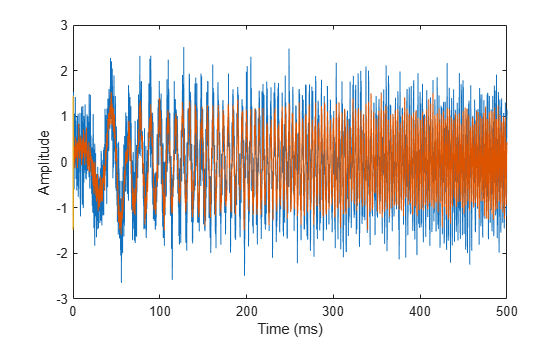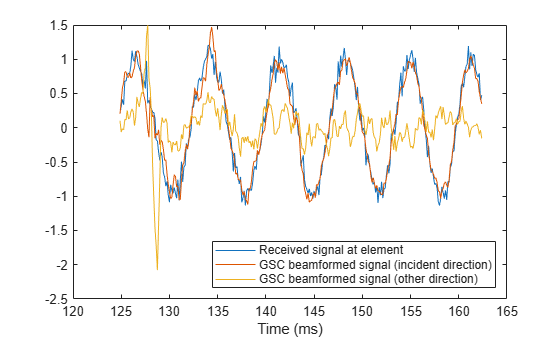# phased.GSCBeamformer

Generalized sidelobe canceler beamformer

## Description

The `phased.GSCBeamformer` System object™ implements a generalized sidelobe cancellation (GSC) beamformer. A GSC beamformer splits the incoming signals into two channels. One channel goes through a conventional beamformer path and the second goes into a sidelobe canceling path. The algorithm first pre-steers the array to the beamforming direction and then adaptively chooses filter weights to minimize power at the output of the sidelobe canceling path. The algorithm uses least mean squares (LMS) to compute the adaptive weights. The final beamformed signal is the difference between the outputs of the two paths.

To compute the beamformed signal:

1. Create the `phased.GSCBeamformer` object and set its properties.

2. Call the object with arguments, as if it were a function.

To learn more about how System objects work, see What Are System Objects?

## Creation

### Syntax

``beamformer = phased.GSCBeamformer``
``beamformer = phased.GSCBeamformer(Name,Value)``

### Description

````beamformer = phased.GSCBeamformer` creates a GSC beamformer System object, `beamformer`, with default property values.```
````beamformer = phased.GSCBeamformer(Name,Value)` creates a GSC beamformer object, `beamformer`, with each specified property Name set to the specified Value. You can specify additional name-value pair arguments in any order as (`Name1`,`Value1`,...,`NameN`,`ValueN`). Enclose each property name in single quotes.Example: ```beamformer = phased.GSCBeamformer('SensorArray',phased.ULA('NumElements',20),'SampleRate',300e3)``` sets the sensor array to a uniform linear array (ULA) with default ULA property values except for the number of elements. The beamformer has a sample rate of 300 kHz.```

## Properties

expand all

Unless otherwise indicated, properties are nontunable, which means you cannot change their values after calling the object. Objects lock when you call them, and the `release` function unlocks them.

If a property is tunable, you can change its value at any time.

For more information on changing property values, see System Design in MATLAB Using System Objects.

Sensor array, specified as a Phased Array System Toolbox array System object. The array cannot contain subarrays.

Example: `phased.URA`

Signal propagation speed, specified as a real-valued positive scalar. Units are in meters per second. The default propagation speed is the value returned by `physconst('LightSpeed')`.

Example: `3e8`

Data Types: `single` | `double`

Sample rate of signal, specified as a positive scalar. Units are in Hz. The System object uses this quantity to calculate the propagation delay in units of samples.

Example: `1e6`

Data Types: `single` | `double`

Length of the signal path FIR filters, specified as a positive integer. This property determines the adaptive filter size for the sidelobe canceling path. The FIR filter for the conventional beamforming path is a delta function of the same length.

Example: `4`

Data Types: `double` | `single`

The adaptive filter step size factor, specified as a positive real-valued scalar. This quantity, when divided by the total power in the sidelobe canceling path, sets the actual adaptive filter step size that is used in the LMS algorithm.

Data Types: `double` | `single`

Source of beamforming direction, specified as `'Property'` or ```'Input port'```. Specify whether the beamforming direction comes from the `Direction` property of this object or from the input argument, `ANG`. Values of this property are:

 `'Property'` Specify the beamforming direction using the `Direction` property. `'Input port'` Specify the beamforming direction using the input argument, `ANG`.

Data Types: `char`

Beamforming directions, specified as a real-valued 2-by-1 vector or a real-valued 2-by-L matrix. For a matrix, each column specifies a different beamforming direction. Each column has the form `[AzimuthAngle;ElevationAngle]`. Azimuth angles must lie between –180° and 180° and elevation angles must lie between –90° and 90°. All angles are defined with respect to the local coordinate system of the array. Units are in degrees.

Example: `[40;30]`

#### Dependencies

To enable this property, set the `DirectionSource` property to `'Property'`.

Data Types: `single` | `double`

## Usage

### Syntax

``Y = beamformer(X)``
``Y = beamformer(X,ANG)``

### Description

example

````Y = beamformer(X)` performs GSC beamforming on the input, `X`, and returns the beamformed output, `Y`.```

example

````Y = beamformer(X,ANG)` uses `ANG` as the beamforming direction. To use this syntax, set the DirectionSource property to `'Input port'`.```

### Input Arguments

expand all

Input signal, specified as a complex-valued M-by-N matrix. M is the signal length and N is the number of array elements specified in the SensorArray property. M must be larger than the length of the filter specified by the FilterLength property.

The size of the first dimension of the input matrix can vary to simulate a changing signal length. A size change can occur, for example, in the case of a pulse waveform with variable pulse repetition frequency.

Data Types: `double` | `single`
Complex Number Support: Yes

Beamforming directions, specified as a real-valued 2-by-1 column vector The vector has the form `[AzimuthAngle;ElevationAngle]`. Units are in degrees. The azimuth angle must lie between –180° and 180°, and the elevation angle must lie between –90° and 90°.

Example: `[40;10]`

#### Dependencies

To enable this argument, set the DirectionSource property to `'Input port'`.

Data Types: `double`

### Output Arguments

expand all

Beamformed output, returned as a complex-valued 1-by-Mvector, where M is the number of rows of the input `X`.

Data Types: `double` | `single`
Complex Number Support: Yes

## Object Functions

To use an object function, specify the System object as the first input argument. For example, to release system resources of a System object named `obj`, use this syntax:

`release(obj)`

expand all

 `step` Run System object algorithm `release` Release resources and allow changes to System object property values and input characteristics `reset` Reset internal states of System object

## Examples

collapse all

Create a GSC beamformer for a 11-element acoustic array in air. A chirp signal is incident on the array at $-5{0}^{\circ }$ in azimuth and ${0}^{\circ }$ in elevation. Compare the GSC beamformed signal to a Frost beamformed signal. The signal propagation speed is 340 m/s and the sample rate is 8 kHz.

Create the microphone and array System objects. The array element spacing is one-half wavelength. Set the signal frequency to the one-half the Nyquist frequency.

```c = 340.0; fs = 8.0e3; fc = fs/2; lam = c/fc; transducer = phased.OmnidirectionalMicrophoneElement('FrequencyRange',[20 20000]); array = phased.ULA('Element',transducer,'NumElements',11,'ElementSpacing',lam/2);```

Simulate a chirp signal with a 500 Hz bandwidth.

```t = 0:1/fs:.5; signal = chirp(t,0,0.5,500);```

Create an incident wave arriving at the array. Add gaussian noise to the wave.

```collector = phased.WidebandCollector('Sensor',array,'PropagationSpeed',c, ... 'SampleRate',fs,'ModulatedInput',false,'NumSubbands',512); incidentAngle = [-50;0]; signal = collector(signal.',incidentAngle); noise = 0.5*randn(size(signal)); recsignal = signal + noise;```

Perform Frost beamforming at the actual incident angle.

```frostbeamformer = phased.FrostBeamformer('SensorArray',array,'PropagationSpeed', ... c,'SampleRate',fs,'Direction',incidentAngle,'FilterLength',15); yfrost = frostbeamformer(recsignal);```

Perform GSC beamforming and plot the beamformer output against the Frost beamformer output. Also plot the nonbeamformed signal arriving at the middle element of the array.

```gscbeamformer = phased.GSCBeamformer('SensorArray',array, ... 'PropagationSpeed',c,'SampleRate',fs,'Direction',incidentAngle, ... 'FilterLength',15); ygsc = gscbeamformer(recsignal); plot(t*1000,recsignal(:,6),t*1000,yfrost,t,ygsc) xlabel('Time (ms)') ylabel('Amplitude')```Zoom in on a small portion of the output.

```idx = 1000:1300; plot(t(idx)*1000,recsignal(idx,6),t(idx)*1000,yfrost(idx),t(idx)*1000,ygsc(idx)) xlabel('Time (ms)') legend('Received signal','Frost beamformed signal','GSC beamformed signal')```Create a GSC beamformer for an 11-element acoustic array in air. A chirp signal is incident on the array at $-5{0}^{\circ }$ in azimuth and ${0}^{\circ }$ in elevation. Compute the beamformed signal in the direction of the incident wave and in another direction. Compare the two beamformed outputs. The signal propagation speed is 340 m/s and the sample rate is 8 kHz. Create the microphone and array System objects. The array element spacing is one-half wavelength. Set the signal frequency to the one-half the Nyquist frequency.

```c = 340.0; fs = 8.0e3; fc = fs/2; lam = c/fc; transducer = phased.OmnidirectionalMicrophoneElement('FrequencyRange',[20 20000]); array = phased.ULA('Element',transducer,'NumElements',11,'ElementSpacing',lam/2);```

Simulate a chirp signal with a 500 Hz bandwidth.

```t = 0:1/fs:0.5; signal = chirp(t,0,0.5,500);```

Create an incident wavefield hitting the array.

```collector = phased.WidebandCollector('Sensor',array,'PropagationSpeed',c, ... 'SampleRate',fs,'ModulatedInput',false,'NumSubbands',512); incidentAngle = [-50;0]; signal = collector(signal.',incidentAngle); noise = 0.1*randn(size(signal)); recsignal = signal + noise;```

Perform GSC beamforming and plot the beamformer outputs. Also plot the nonbeamformed signal arriving at the middle element of the array.

```gscbeamformer = phased.GSCBeamformer('SensorArray',array, ... 'PropagationSpeed',c,'SampleRate',fs,'DirectionSource','Input port', ... 'FilterLength',5); ygsci = gscbeamformer(recsignal,incidentAngle); ygsco = gscbeamformer(recsignal,[20;30]); plot(t*1000,recsignal(:,6),t*1000,ygsci,t*1000,ygsco) xlabel('Time (ms)') ylabel('Amplitude') legend('Received signal at element','GSC beamformed signal (incident direction)', ... 'GSC beamformed signal (other direction)','Location','southeast')```Zoom in on a small portion of the output.

```idx = 1000:1300; plot(t(idx)*1000,recsignal(idx,6),t(idx)*1000,ygsci(idx),t(idx)*1000,ygsco(idx)) xlabel('Time (ms)') legend('Received signal at element','GSC beamformed signal (incident direction)', ... 'GSC beamformed signal (other direction)','Location','southeast')```## Algorithms

expand all

 Griffiths, L. J., and Charles W. Jim. "An alternative approach to linearly constrained adaptive beamforming." IEEE Transactions on Antennas and Propagation, 30.1 (1982): 27-34.

 Van Trees, H. Optimum Array Processing. New York: Wiley-Interscience, 2002.

 Johnson, D.H., and Dan E. Dudgeon, Array Signal Processing, Englewood Cliffs: Prentice-Hall, 1993.